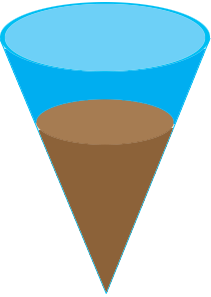SEARCH HOMEMath Central Quandaries & QueriesQuestion from Janice, a teacher: A cone with a radius of 3.5 cm and a height of 12 cm is 2/3 full of sand. What is the volume of the sand inside? I think it is 1/3(Pi x 3.5^2)(12)(2/3)^3 = 45 cubic cm I was told that since only the height was changed that the scale factor 2/3 was only used as a factor one time. I was told the answer was 101 cubic cm. I don't think you can change the height of a cone without proportionally changing the radius. You can change the height of a cylinder or a prism and the size of the base will remain the same, but this does not work on a cone. Can you please tell me who is correct? Thank you so much for your time.Hi Janice,

The volume of the conical container is

$\frac13 \; \pi \times 3.5^2 \times 12 = 154 \mbox{ cubic centimeters.}$

The container is $\frac23$ full of sand so the volume of sand in the container is two thirds of 154 cubic centimeters or $\frac23 \times 154$ cubic centimeters.

I hope this helps,
Penny

Janice wrote back

Thank you so much for answering my question. I was looking at the original height of the cone of 12 cm, and I was taking 2/3 of 12 to get the new height of 8. The new height is not 8, and we don't care what the new height is. We are only taking 2/3 of the original volume. I have been worried about this question for more than a week. In 8th grade math we teach dimensional change. I was trying to make this a dimensional change problem. If we were taking 2/3 of the height, I would be correct. My district coordinator tried to explain this to me. She was so patient. I just did not get it.

Janice,

The conical container and the cone of sand are similar figures.With your interpretation, since the height of the sand is 2/3 the height of the container it must be that the radius of the surface of the sand is 2/3 the radius of the top of the container. Thus the volume of sand is

$\frac13 \; \pi \; \left(\frac23 \times 3.5\right)^2 \times \left(\frac23 \times 12\right) \mbox{ cubic centimeters.}$

Exactly what you have.

PennyMath Central is supported by the University of Regina and the Imperial Oil Foundation.